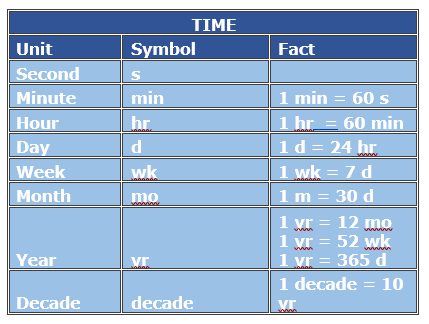# Introduction to Adding Time

#### Complete Python Prime Pack

9 Courses     2 eBooks

#### Artificial Intelligence & Machine Learning Prime Pack

6 Courses     1 eBooks

#### Java Prime Pack

9 Courses     2 eBooks

The basic metric unit for time is a second. Other metric units for time are minutes, hours, days and so on. These units of time are same as the US customary units of time.

In this lesson, we solve problems on addition among various metric units for time.

The table below helps in conversion among various metric units for time.Add the following times in hours and minutes.

4 hours 15 minutes + 2 hours 20 minutes =_________

### Solution

Step 1:

We know that 1 hour = 60 minutes

Adding the given times

4 hours 15 minutes + 2 hours 20 minutes =

4 hours + 2 hours + 15 minutes + 20 minutes =

6 hours + 35 minutes = 6 hours 35 minutes

Step 2:

So, the sum is = 6 hours 35 minutes

Add the following times in hours and minutes.

1 hour 30 minutes + 5 hours 35 minutes =_________

### Solution

Step 1:

We know that 1 hour = 60 minutes

Adding the given times

1 hour 30 minutes + 5 hours 35 minutes =

1 hours + 5 hours + 30 minutes + 35 minutes =

6 hours + 65 minutes =

6 hours + 1 hour + 5 minutes = 7 hours 5 minutes

(as 60 minutes = 1 hour)

Step 2:

So, the sum is = 7 hours 5 minutes

Add the following times in hours and minutes.

7 hours 20 minutes + 3 hours 50 minutes =_________

### Solution

Step 1:

We know that 1 hour = 60 minutes

Adding the given times

7 hours 20 minutes + 3 hours 50 minutes =

7 hours + 3 hours + 20 minutes + 50 minutes =

10 hours + 70 minutes =

10 hours + 1 hour + 10 minutes = 11 hours 10 minutes

(as 60 minutes = 1 hour)

Step 2:

So, the sum is = 11 hours 10 minutes